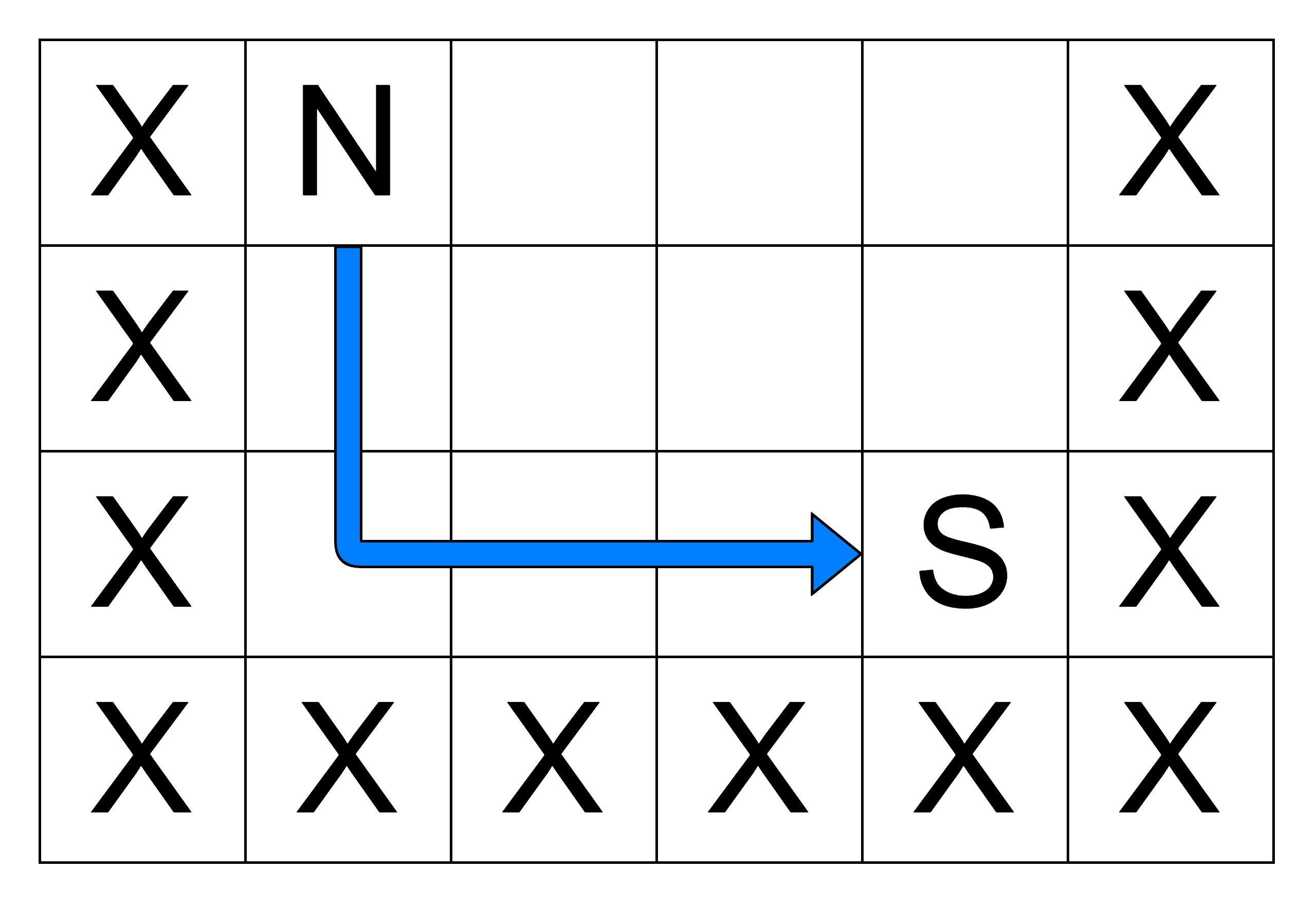# Path to the nearest service station

Posted: 26 Mar, 2021
Difficulty: Moderate

## PROBLEM STATEMENT

#### The Ninja can only travel to adjacent free cells from his current location, i.e., in the North, South, East, or West direction. Your task is to find the length of the shortest path through which the Ninja can get to a service station. If there is no such path available, return -1.

##### Example:
``````‘N’ = 4, ‘M’ = 6
Given ‘GRID’:
(Empty cells represent the character ‘O’)
````````````The Ninja can reach the nearest service station by moving two cells south and then three cells east. So, the answer is ‘5’.
``````
##### Input format:
``````The first line of input contains an integer ‘T’ which denotes the number of test cases. Then, the ‘T’ test cases follow.

Each test case’s first line contains two space-separated integers, ‘N’ and ‘M’, denoting the number of rows and columns in the ‘GRID’.

The next ‘N’ lines contain ‘M’ characters denoting the elements of the 'GRID'.
``````
##### Output format:
``````For every test case, return the length of the shortest path to a service station. If no such path is available, return -1;
``````
##### Note:
``````You do not need to print anything; it has already been taken care of. Just implement the function.
``````
##### Constraints:
``````1 <= T <= 10
1 <= N, M <= 100
Value in each element of ‘GRID’ = {‘N’, ‘S’, ‘O’, ‘X’}

Time limit: 1 second
``````Approach 1

Consider the given ‘GRID’ as an undirected graph where each cell represents a vertex, and if two free cells are adjacent, they are connected by an edge. Dijkstra’s algorithm is used for finding the shortest path between nodes in a weighted graph. As the given graph is unweighted, the path length is equal to the number of edges, so consider each edge’s weight to be ‘1’. Consider the cell with the ninja as the source node and break the algorithm when we reach a cell with a service station. There are ‘N x M’ vertices where each vertex is of the form ‘[r, c]’ (‘r’: row number, and ‘c’: column number). Below is an implementation of Dijkstra’s algorithm using priority queue(in this case: min-heap):

First find the presence of ninja:

• Initialize two integers ‘SOURCEROW = -1’ and ‘SOURCECOL = -1’. Use them to store the location of the cell where the ninja is present.
• Run a loop where ‘i’ ranges from ‘0’ to ‘N - 1’:
• Run a loop where ‘j’ ranges from ‘0’ to ‘M - 1’:
• If ‘GRID[i][j]’ is equal to ‘N’:
• ‘SOURCEROW = i’ and ‘SOURCECOL = j’
• Break the loop.
• If ‘SOURCEROW ’ is not equal to ‘-1’:
• Break the loop. We have found the cell with the ninja.

Now apply Dijkstra’s algorithm to find the minimum cost path to reach the target:

• Initialize 2-d array ‘path[n][m]’ with value ‘INT_MAX’. Use ‘PATH[i][j]’ to store the path length for cell ‘[i, j]’.
• Set ‘PATH[SOURCEROW ][SOURCERCOL] = 0’ (Length of the path to source vertex is zero).
• Create a min priority queue ‘MINPATH’ that stores ‘CELL’ objects and orders them by ‘CELL -> PATH’. A ‘CELL’ contains three values {‘PATH’, ‘ROW’, ‘COL’}.
• Insert {0, SOURCEROW , SOURCECOL}  in ‘MINPATH’. The value ‘PATH’ for the source vertex is zero.
• Initialize ‘DIR = [(-1, 0), (0, 1), (1, 0), (0, -1)]. Add ‘DIR[i]’ to a cell to move to an adjacent cell in the direction: North, east, south or west.
• Run a loop until ‘MINPATH’ is not empty:
• ‘CUR = MINPATH.pop()’. Get the vertex with the smallest known path length and remove it from ‘MINPATH’.
• ‘ROW = CUR →ROW’ (Current row).
• ‘COL = CUR →COL’ (Current column).
• If ‘PATH[ROW][COL] < (CUR→PATH)’ then, ignore this instance of the cell as it doesn’t store the minimum path length:
• Continue the loop.
• If ‘GRID[ROW][COL]’ is equal to ‘S’, then this is the nearest service station:
• Return ‘CUR→PATH’ as the answer.
• Run a loop where ‘i’ ranges from 0 to 3:
• ‘NEWROW = ROW + DIR[i]’. ‘NEWROW’ is the row no. of the adjacent cell.
• ‘NEWCOL = COL + DIR[i]’. ‘NEWCOL’ is the column no. of the adjacent cell.
• If ‘NEWROW >= N’ or ‘NEWROW < 0’ or ‘NEWCOL >= M’ or ‘NEWCOL < 0’ or  ‘GRID[NEWROW ][NEWCOL ]’ is equal to ‘X’ , then the adjacent cell is not a valid cell:
• Continue the loop.
• If ‘PATH[NEWROW ][NEWCOL ] > PATH[ROW][COL] + 1’, then:
• ‘PATH[NEWROW ][NEWCOL ] = PATH[ROW][COL] + 1’
• ‘MINPATH.push({path[NEWROW ][NEWCOL ], NEWROW , NEWCOL }).
• Return ‘-1’ as the answer as we cannot reach any cell with a service station.